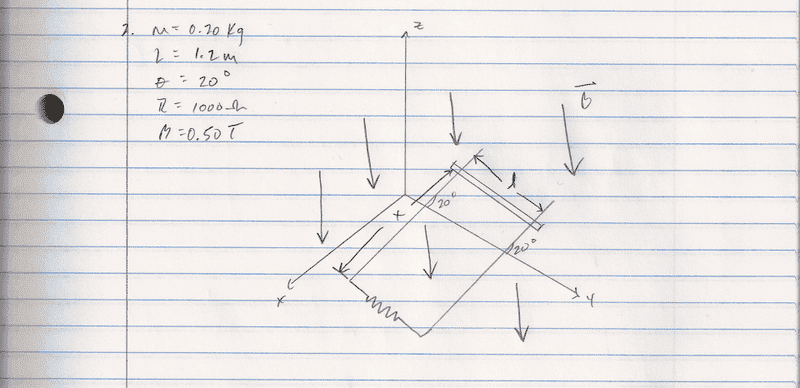# Motional EMF

Robb

## Homework Statement## Homework Equations## The Attempt at a Solution

Kinetic Energy + Potential energy=0

1/2mv^2 = mgcos(20)
100v^2 = 1.96cos(20)
v^2 = .0184
v = .136 m/s

No idea if this is correct or if I should proceed with energy considerations. Please advise.

## Answers and Replies

Homework Helper
Gold Member
Under the influence of gravity and the normal force of the incline, the resulting motion is not at constant velocity. Right?

There must be an additional force somewhere to balance the forces.
So, you are missing something.
Is there anything else given in the problem that can be used?

Robb
Thanks for the response. I got help in class last night!